# 深入浅出的模型压缩：你一定从未见过如此通俗易懂的 Slimming 操作

## 目录

• 如何衡量模型的复杂度？

• Literature Review for Model Compression

• Slimming

• Slimming操作压缩GAN模型

• 参考文献

## 如何衡量模型的复杂度？

• model size
• Runtime Memory
• Number of computing operations

• model size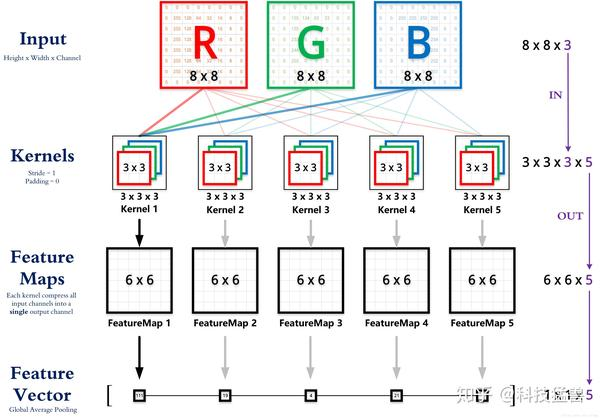$$Params{w}=K{in}\times K{out}\times C{in}\times C_{out}\tag{1}$$

$$Params{b}=C{out}\tag{2}$$

$$Params=K{in}\times K{out}\times C{in}\times C{out}+C_{out}\tag{3}$$

$$=(K{in}\times K{out}\times C{in}+1)\times C{out}$$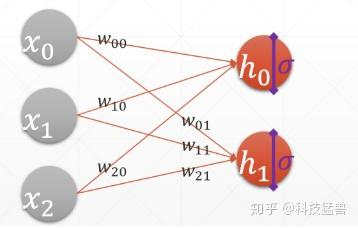$$Params{w}=N{in}\times N_{out}\tag{4}$$

$$Params{b}=N{out}\tag{5}$$

$$Params{}=N{in}\times N{out}+N{out}\tag{6}$$

• Run time Memory

• Number of computing operations

$$FLOPs|{bias}=2K{in}\times K{out}\times C{in}\times C{out}\times H{out}\times W_{out}\tag{1}$$

$$FLOPs|{no bias}=(2K{in}\times K{out}\times C{in}-1)\times C{out}\times H{out}\times W_{out}\tag{2}$$

$(K{in}\times K{out}\times C{in}-1)\times C{out}\times H{out}\times W{out}$ 为加法的运算量。

$1\times C{out}\times H{out}\times W_{out}$ 为 $bias$ 的运算量。

$$FLOPs{}=(2N{in}-1)N_{out}\tag{8}$$

$N{in}N{out}$ 为乘法的运算量， $(N{in}-1)N{out}$ 为加法的运算量。

MACs与FLOPs的关系：

y = w*x + w*x + w*x + ... + w[n8]*x

$$MACC{}=N{in}\times N_{out}\tag{9}$$

$$y = max(x, 0)\tag{10}$$

$$y = \frac{1}{1+e^{-x}}\tag{11}$$

$$K × K × C{in} × C{out}× H{out} × W{out} \tag{12}$$

https://zhuanlan.zhihu.com/p/268816646

## Literature Review for Model Compression：

1 剪枝就是通过去除网络中冗余的channels,filters, neurons, or layers以得到一个更轻量级的网络，同时不影响性能。 代表性的工作有：

(NIPS 2015)： Learning both weights and connections for efficient neural network

LASSO regression(ICCV 2017)： Channel pruning for accelerating very deep neural networks
Discriminative channels(NIPS 2018)： Discrimination-aware channel pruning for deep neural networks

neuron level sparsity(ECCV 2017)： Less is more: Towards compact cnns
Structured Sparsity Learning(NIPS 2016)： Learning structured sparsity in deep neural networks

2 轻量化模块设计就是设计一些计算效率高，适合在端侧设备上部署的模块。 代表性的工作有：

Bottleneck(ICLR 2017)： Squeezenet: Alexnet-level accuracy with 50x fewer parameters and 0.5 mb model size
MobileNet(CVPR 2017)： Mobilenets: Efficient convolutional neural networks for mobile vision applications
ShuffleNet(CVPR 2018)： Shufflenet: An extremely efficient convolutional neural network for mobile devices
SE模块(CVPR 2018)： Squeeze-and-excitation networks

Shift操作填坑(Arxiv)： Shift-based primitives for efficientconvolutional neural networks

GhostNet(CVPR 2020)： GhostNet: More features from cheap operations

3 蒸馏就是过模仿教师网络生成的软标签将知识从大的，预训练过的教师模型转移到轻量级的学生模型。 代表性的工作有：

Hinton(NIPS 2015)： Distilling the knowledge in a neural network

4 量化就是减少权重等的表示的位数，比如原来网络权值用32 bit存储，现在我只用8 bit来存储，以减少模型的Memory为原来的 $\frac{1}{4}$ ；更有甚者使用二值神经网络。 代表性的工作有：

(ICML 2015)： Compressing neural networks with the hashing trick
(NIPS 2015)： Learning both weights and connections for efficient neural network
(CVPR 2018)： Quantization and training of neural networks for efficient integer-arithmetic-only inference
(CVPR 2016)： Quantized convolutional neural networks for mobile devices
(ICML 2018)： Deep k-means: Re-training and parameter sharing with harder cluster assignments for compressing deep convolutions
(CVPR 2019)： Learning to quantize deep networks by optimizing quantization intervals with task loss
(CVPR 2019)： HAQ: Hardware-Aware automated quantization with mixed precision.

Binarized weights(NIPS 2015)： BinaryConnect: Training deep neural networks with binary weights during propagations
Binarized activations(NIPS 2016)： Binarized neural networks
XNOR(ECCV 2016)： Xnor-net: Imagenet classification using binary convolutional neural networks
more weight and activation(NIPS 2017)： Towards accurate binary convolutional neural network
(ECCV 2020)： Learning Architectures for Binary Networks
(ECCV 2020)： BATS: Binary ArchitecTure Search

5 低秩分解就是将原来大的权重矩阵分解成多个小的矩阵，而小矩阵的计算量都比原来大矩阵的计算量要小。 代表性的工作有：

6 加法网络就是：利用卷积所计算的互相关性其实就是一种“相似性的度量方法”，所以在神经网络中用加法代替乘法，在减少运算量的同时获得相同的性能。 代表性的工作有：

(CVPR 20)： AdderNet: Do We Really Need Multiplications in Deep Learning\?
(NIPS 20)： Kernel Based Progressive Distillation for Adder Neural Networks
(Arxiv)： AdderSR: Towards Energy Efficient Image Super-Resolution

7 Slimming：

(ICCV 2017)：清华张长水，黄高团队： Learning Efficient Convolutional Networks through Network Slimming
(ECCV 2020)：得克萨斯大学奥斯汀分校团队： GAN Slimming: All-in-One GAN Compression by A Unified Optimization Framework

## Slimming：

1. 只能解决上面讲的3个指标(model size, runtime memory, number of computation numbers)中的1-2个，即比如剪枝减少了模型参数量，可是运行时占得内存空间仍旧很大因为许多Memory花在了activation map上面，而不是weights上面，另外有的pruning方法需要专门的硬件电路；或是比如量化可以减少model size，可是运行时占得内存空间仍旧很大，Latency也没有下降，因为你得把权重还原回去。这是第一个缺点。
2. 许多技术依赖于specially designed software/hardware accelerators，即如果我想用这个方法，丫还得专门去买你们设计的硬件电路芯片或者对应的软件[○･｀Д´･ ○]。有一个组的很多文章都是这样。换句话说，以上这些压缩方法不够general-purpose

weight-level往往需要特殊的硬件加速器或软件来实现fast inference。

layer-level只适用于一些特别深的模型，比如超过50层的模型，但是不是很灵活。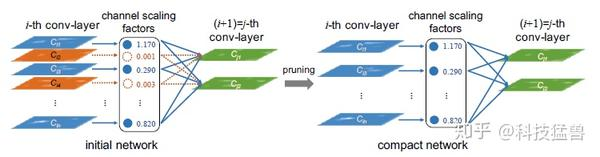slimming的具体做法

$$L=\sum{(x,y)}^{}{l(f(x,W),y)+\lambda \sum{r \in \Gamma}{g(\gamma)}}\tag{13}$$

$$\hat z= \frac{z_{in}-\mu _B}{\sqrt{\sigmaB^2+\epsilon}};z{out}=\gamma \hat z +\beta\tag{14}$$

1. 如果我们放弃BN而给模型每一层加上一个scaling层：那么这个scaling层的参数将会是无意义的。因为convolution层和scaling层都是线性变换(Linear Transformation)，你用这个scaling层，和把卷积层的weights调大一点结果是一样的。
2. 如果我们在BN层之前加上一个scaling层：那么 $\gamma$ 带来的scaling的效应将会被BN层的normalization的作用抹去。
3. 如果我们在BN层之后加上一个scaling层：那么每个channel将会有2个scaling factor，没有必要。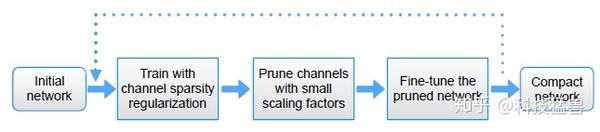Slimming操作流程图，Slimming一时爽，一直Slimming一直爽。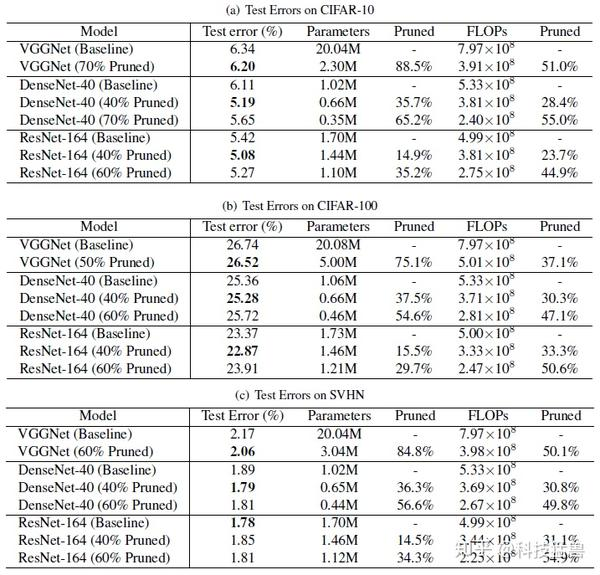Slimming操作的实验结果

Baseline是指不使用sparsity regularization进行训练。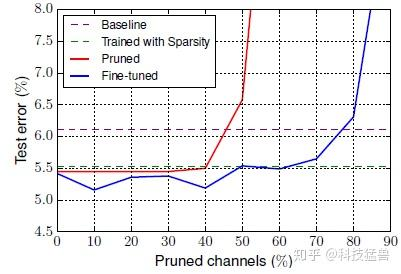• 当剪枝率超过一定的阈值时，精度会大大降低。
• Finetuning过程可以补偿精度的下降。
• 当使用sparsity罚项时，即使不fine-tuning，性能也会优于baseline。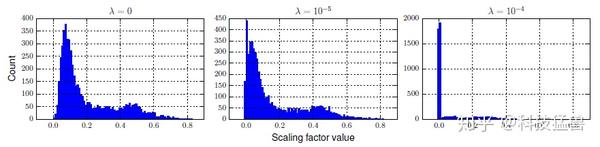Distributions of scaling factors in a trained VGGNet under various degree of sparsity regularization

## Slimming操作压缩GAN模型

ICLR 2016 Best Paper： 韩松老师的Deep compression: Compressing deep neural networks with pruning, trained quantization and huffman coding

• end-to-end：端到端
• unified：统一的框架
• combining multiple compression means：包含多种压缩方法

$$\min{G}\max{D} L{GAN},~\text{where } L{GAN}=\exp{y\in Y}[\text{log}(D(y))] + \exp{x\in X}[\text{log}(1-D(G(x))]\tag{15}$$

$$L{dist} = E{x\in X} [\textrm{d}(G(x), G_0(x))]\tag{16}$$

$$L{dist}(W,\gamma) + \rho L{cp}(\gamma) = E_{x\in X} [\textrm{d}(G(x;W,\gamma), G_0(x))] + \rho |\gamma|_1\tag{17}$$

$$L(W,\gamma,\theta) = L{GAN}(W,\gamma,\theta) + \beta L{dist}(W,\gamma) + \rho L{cp}(\gamma)\ \ = E{y\in Y}~[\text{log}(D(y;\theta))] + E_{x\in X}~[ \text{log}(1-D({\color{red}{G_q}}(x;{\color{red}{qw}}(W),\gamma);\theta))] \ \ = E{x\in X}~[{\color{blue}{\beta\textrm{d}(}}{\color{red}{G_q}}(x;{\color{red}{q_w}}(W),\gamma), G_0(x){\color{blue})}] + {\color{green}{\rho|\gamma|_1}}\tag{18}$$

$$\min{W,\gamma}\max{\theta}~L(W,\gamma,\theta)\tag{19}$$

1. 每一步操作可解释。
2. 轻量化，稳定。
3. 不需要NAS方法耗费大量的计算资源与精心设计搜索空间。

• minmax优化本身就是不稳定的。
• 更新 $G$ 的权重$W$ 会遇到不可微分的量化参数，如何应用梯度下降？
• 更新罚项参数 $\gamma$ ，罚项是 $L_1$ 范数，也是不可微的，如何应用梯度下降？

19式中包含 $W$ 的只有前两项，因此这个问题可以被等效为：

$$\min_{W}~L_W(W), \ \text{where}~LW(W) = \exp{x\in X}[\text{log}(1-D(G_q(x;q_w(W),\gamma);\theta)) \ \hspace{10em} + \beta \textrm{d}(G_q(x;q_w(W),\gamma), G_0(x))]\tag{20}$$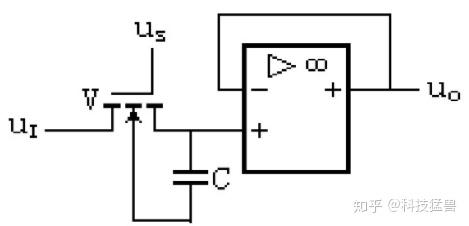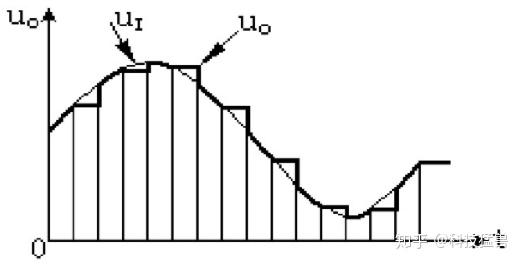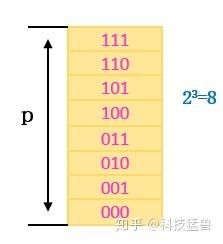$$q_a(a) = \text{round}(\min(\max(0, a), p) / s_a) \cdot s_a\tag{21}$$

$$q_w(w) = \text{round}(w / s_w) \cdot s_w\tag{22}$$

$${\partial q_a(a) \over \partial a} = \begin{cases} 1 \quad &\text{if 0 \leq a \leq p};\ 0 &\text{otherwise}. \end{cases}\tag{23}$$

$${\partial q_w(w) \over \partial w} = 1\tag{24}$$

19式中包含 $\gamma$ 的项保留，因此这个问题可以被等效为：

$$\min{\gamma} L{\gamma}(\gamma) + \rho|\gamma|1, \ \text{where}~L{\gamma}(\gamma) = \exp_{x\in X}[\text{log}(1-D(G_q(x;q_w(W),\gamma);\theta)) \ \hspace{6em} + \beta \textrm{d}(G_q(x;q_w(W),\gamma), G_0(x))]\tag{25}$$

$$g{\gamma}^{(t)} \gets \nabla{\gamma} L{\gamma}(\gamma)\bigg\rvert{\gamma=\gamma^{(t)}}\tag{26}$$

$$\gamma^{(t+1)} \gets \textrm{prox}{\rho\eta^{(t)}}(\gamma^{(t)}-\eta^{(t)}g{\gamma}^{(t)})\tag{27}$$

$$\textrm{prox}_\lambda(\text{x}) = \textrm{sgn}(\text{x}) \odot \textrm{max}(|\text{x}|-\lambda\mathbf{1}, \mathbf{0})\tag{28}$$

https://zhuanlan.zhihu.com/p/277041051

19式中包含 $\theta$ 的项保留，因此这个问题可以被等效为：

$$\max{\theta}~L\theta(\theta), \ \text{where}~L\theta(\theta) = \exp{y\in Y}[\text{log}(D(y;\theta))] + \exp_{x\in X}[\text{log}(1-D(G_q(x);\theta))]\tag{29}$$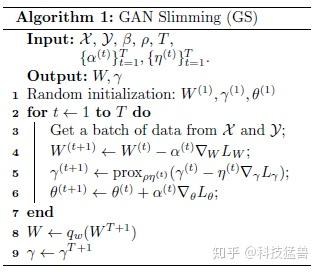GAN Slimming算法流程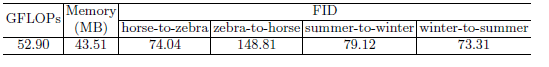CycleGAN原模型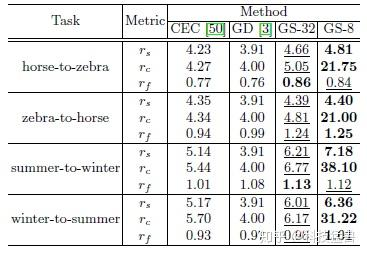CycleGAN压缩模型

$$rc = \frac{\textrm{ModelSize}{G0}}{\textrm{ModelSize}{G}}, ~rs = \frac{\textrm{FLOPs}{G_0}}{\textrm{FLOPs}_G}, ~rf = \frac{\textrm{FID}{G_0}}{\textrm{FID}_G}\tag{30}$$

GS-32就是不进行量化的32位存储。GS-8就是Slimming操作完成的8位存储的结果。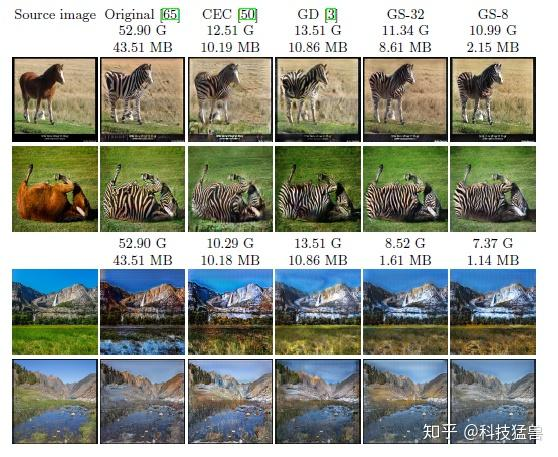GAN Slimming可视化实验结果

D：Distillation：只使用蒸馏技术，来自1的方法。

CEC：来自2的方法。

D+CP：Distillation与channel pruning一起用。

CP+D：channel pruning与Distillation一起用。

GS-8 (MSE)：GS-8 换成MSE Loss。

PostQ：GS-32+Quantization：先使用GS-32，再使用8 bit量化和fin-tune。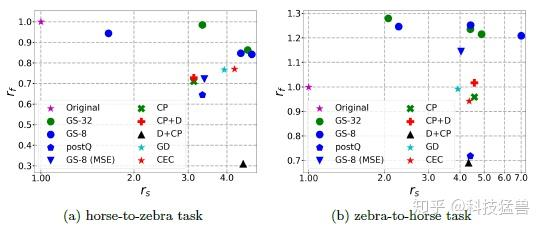Distilling portable Generative Adversarial Networks for Image Translation

 Co-Evolutionary Compression for Unpaired Image Translation

 Convex Optimization, Lecture 7, CMU

 Learning Efficient Convolutional Networks through Network Slimming

 GAN Slimming: All-in-One GAN Compression by A Unified Optimization Framework

 Proximal Gradient Descent (by Ryan Tibshirani from CMU)：http://stat.cmu.edu/\~ryantibs/